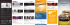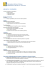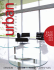# Interpreting Algebraic Expressions

## Transcription

Interpreting Algebraic Expressions
```CONCEPT DEVELOPMENT
Mathematics Assessment Project
CLASSROOM CHALLENGES
A Formative Assessment Lesson
Interpreting Algebraic
Expressions
Mathematics Assessment Resource Service
University of Nottingham & UC Berkeley
For more details, visit: http://map.mathshell.org
© 2015 MARS, Shell Center, University of Nottingham
May be reproduced, unmodified, for non-commercial purposes under the Creative Commons license
detailed at http://creativecommons.org/licenses/by-nc-nd/3.0/ - all other rights reserved
Interpreting Algebraic Expressions
MATHEMATICAL GOALS
This lesson unit is intended to help you assess how well students are able to translate between words,
symbols, tables, and area representations of algebraic expressions. It will help you to identify and
support students who have difficulty:
• Recognizing the order of algebraic operations.
• Recognizing equivalent expressions.
• Understanding the distributive laws of multiplication and division over addition (expansion of
parentheses).
COMMON CORE STATE STANDARDS
This lesson relates to the following Standards for Mathematical Content in the Common Core State
Standards for Mathematics:
A-SSE: Interpret the structure of expressions.
This lesson also relates to the following Standards for Mathematical Practice in the Common Core
State Standards for Mathematics, with a particular emphasis on Practices 1, 2, and 7:
1. Make sense of problems and persevere in solving them.
2. Reason abstractly and quantitatively.
4. Model with mathematics.
5. Use appropriate tools strategically.
6. Attend to precision.
7. Look for and make use of structure.
8. Look for and express regularity in repeated reasoning.
INTRODUCTION
The lesson unit is structured in the following way:
• Before the lesson, students work individually on an assessment task designed to reveal their
current understanding and difficulties. You then review their work and formulate questions for
students to answer, to help them improve their solutions.
• During the lesson, students work in pairs or threes to translate between word, symbol, table of
values, and area representations of expressions.
• In a whole-class discussion, students find different representations of expressions and explain
MATERIALS REQUIRED
•
•
•
Each student will need two copies of Interpreting Expressions, a mini-whiteboard, pen, and
eraser.
Each pair of students will need glue, a felt-tipped pen, a large sheet of poster paper, and cut-up
copies of Card Set A: Expressions, Card Set B: Words, Card Set C: Tables, and Card Set D:
Areas. Note that the blank cards are part of the activity.
If you think you will need to continue with the activities into a second lesson, provide envelopes
and paper clips for storing matched cards between lessons.
TIME NEEDED
10 minutes for the assessment task, a 90-minute lesson (or two 50-minute lessons), and 10 minutes in
a follow-up lesson. All timings are approximate and will depend on the needs of the class.
Teacher guide
Interpreting Algebraic Expressions
T-1
BEFORE THE LESSON
Assessment task: Interpreting Expressions (10 minutes)
Have students do this task in class or for
Interpreting Expressions
homework a day or more before the formative
1. Write algebraic expressions for each of the following:
assessment lesson. This will give you an
a. Multiply n by 5 then add 4.
opportunity to assess the work and to find out
b. Add 4 to n then multiply by 5.
the kinds of difficulties students have with it.
c. Add 4 to n then divide by 5.
You should then be able to target your help
d. Multiply n by n then multiply by 3.
more effectively in the subsequent lesson.
e. Multiply n by 3 then square the result.
Give each student a copy of Interpreting
Expressions.
2. The equations below were created by students who were asked to write equivalent expressions on
either side of the equals sign.
Imagine you are a teacher. Your job is to decide whether their work is right or wrong. If you see an
equation that is false, then:
I want you to spend ten minutes working
a. Cross out the expression on the right and replace it with an expression that is equivalent to the
one on the left.
b. Explain what is wrong, using words or diagrams.
Don’t worry too much if you can’t
understand or do everything. There will be
a lesson [tomorrow] with a similar task
!
It is important that, as far as possible, students
are allowed to answer the questions without
assistance.
2(n + 3) = 2n + 3
10n " 5
5
= 2n " 1
(5n )2 = 5n 2
!
If students are struggling to get started, ask
!
them questions that help them to understand
what is required, but do not do the task for them.
!
(n + 3)2 = n 2 + 32 = n 2 + 9
Student Materials
Interpreting Algebraic Expressions
© 2012 MARS, Shell Center, University of Nottingham
S-1
Assessing students’ responses
current levels of understanding. The purpose of doing this is to forewarn you of the difficulties
students will experience during the lesson itself, so that you may prepare carefully.
We suggest that you do not score students’ papers. The research shows that this will be
counterproductive, as it will encourage students to compare their scores and distract their attention
from what they can do to improve their mathematics.
Instead, help students to make further progress by summarizing their difficulties as a list of questions.
Some suggestions for these are given in the Common issues table on the next page. We suggest that
you make a list of your own questions, based on your students’ work, using the ideas on the following
page. We recommend you either:
•
write one or two questions on each student’s work, or
give each student a printed version of your list of questions and highlight the questions relevant to
each student.
If you do not have time to do this, you could select a few questions that will be of help to the majority
of students and write these on the board when you return the work to the students in the follow-up
lesson.
•
Teacher guide
Interpreting Algebraic Expressions
T-2
Common issues:
Suggested questions and prompts:
Writes expressions left to right, showing little
understanding of the order of operations
implied by the symbolic representation
For example: The student writes:
Q1a.
Q1b.
Q1c.
Q1d.
n × 5 + 4 (not incorrect).
4 + n × 5.
4 + n ÷ 5.
n × n × 3.
Does not construct parentheses correctly or
expands them incorrectly
For example: The student writes:
Q1b.
4 + n × 5 instead of 5(n + 4).
Q1c.
4 + n ÷ 5 instead of
4+ n
.
5
Or: The student counts:
Q2.
2(n + 3) = 2n + 3 as correct.
€
Q2.
(5n)2 = 5n2 as correct.
Q2.
(n + 3)2 = n2 + 32 as correct.
€
Identifies errors but does not give explanations
€
For example: The student corrects the first, third,
€and fourth statements, but no explanation or
diagram is used to explain why they are incorrect
(Q2).
• Can you write answers to the following?
4+1×5
4+2×5
4+3×5
calculator. How is your calculator working
these out?
• So what does 4 + n × 5 mean?
Is this the same as Q1b?
• Which one of the following is the odd one out:
Think of a number, add 3, and then multiply
Think of a number, multiply it by 2, and then
Think of a number, multiply it by 2, and then
Why?
• How would you write expressions for these
areas?
n
3
n
3
1
2
2
n n n n n
n n n n n
n
n
n
n
n
n
n
n
3
3
n
n
3
3
• Can you do this in different ways?
Teacher guide
Interpreting Algebraic Expressions
T-3
SUGGESTED LESSON OUTLINE
Interactive whole-class introduction (10 minutes)
Give each student a mini-whiteboard, pen, and eraser and hold a short question and answer session. If
students show any incorrect answers, write the correct answer on the board and discuss any problems.
On your mini-whiteboards, show me an algebraic expression that means:
4n + 3
4(3+ n)
€
€
€
n+5
3
5n2
(5n)2
€
Collaborative activity 1: matching expressions and words
(20 minutes)
The first activity is designed to help students interpret symbols and realize that the way the symbols
€
are written defines the order of operations.
Organize students into groups of two or three.
Display Slide P-1 of the projector resource:
Matching Expressions and Words
4(n +2)
5&.(/0.1#!#21#(3%)#(*+,#6""#\$%&'4#
2(n + 4)
!""#\$%&'#(%#!)#(*+,#-&.(/0.1#21#(3%4#
4n + 2
!""#(3%#(%#!)#(*+,#-&.(/0.1#21#\$%&'4#
Note that one of the algebraic expressions does not have a match in words. This is deliberate! It is to
Projector Resources
Interpreting Algebraic Expresssions
P-1
Model the activity briefly for students, using the examples on the projector resource:
I am going to give each group two sets of cards, one with expressions written in algebra and the
other with words.
Take turns to choose an expression and find the words that match it. [4(n + 2) matches ‘Add 2 to
n then multiply by 4’; 2(n + 4) matches ‘Add 4 to n then multiply by 2’.]
When you are working in groups, you should place these cards side by side on the table and
explain how you know that they match.
If you cannot find a matching card, then you should write your own using the blank cards
provided. [4n + 2 does not match any of the word cards shown on Slide P-1. The word card
‘Multiply n by two, then add four’ does not match any of the expressions.]
Give each small group of students a cut-up copy of Card Set A: Expressions and Card Set B: Words:
Teacher guide
Interpreting Algebraic Expressions
T-4
Card Set B: Words
Card Set A: Expressions
E1
E2
n+6
2
3n
E3
2(n + 3)
!
E7
(3n)
!
E9
2
! E11
!
W3
n +12n + 36
!
2
n +6
E13
W6
Add three to n then multiply by
two.
2
Divide n by two
W10
E12
n +6
W8
W9
n
3+
2
2
Add six to n then square the
Multiply n by two
Square n, then multiply by nine
W11
W12
W13
W14
2
! E14
!
Add six to n then divide by two.
W7
(n + 6)
! E10
W4
W5
n
+6
2
E8
2
Multiply n by three,
then multiply by two.
2n + 6
! E6
! E5
W2
Multiply n by two, then add six.
E4
2n +12
!
W1
2
!
Student materials
Interpreting Algebraic Expressions
© 2014 MARS, Shell Center, University of Nottingham
S-2
Support students in making matches and explaining their decisions. As they do this, encourage them
to speak the algebraic expressions out loud. Students may not be used to ‘talking algebra’ and may
not know how to say what is written, or may do so in ways that create ambiguities.
Student materials
Interpreting Algebraic Expressions
© 2014 MARS, Shell Center, University of Nottingham
S-3
For example, the following conversation between a teacher and student is fairly typical:
Teacher: Tell me in words what this one says. [Teacher writes: 3+
Student:
Three add n divided by two.
Teacher: How would you read this one then? [Teacher writes:
Student:
n
.]
2
(3+ n)
.]
2
Three add n divided by two. Oh, but in the second one you are dividing it all by two.
Teacher: So can you try reading the first one again, so it sounds different from the second one?
Student:
Three add … [pause] … n divided by two [said quickly]. Or n divided by two, then
Students will need to make word cards to match E10: 3+
n
and E12: n2 + 62 .
2
They will also need to make expression cards to match W3: Add 6 to n, then multiply by 2 and W10:
Square n, then multiply by 9.
€
€
Some students may notice that some expressions are equivalent, for example 2(n + 3) and 2n + 6. You
do not need to comment on this now as when Card Set C: Tables is given out, students will be able to
notice this for themselves.
Teacher guide
Interpreting Algebraic Expressions
T-5
Collaborative activity 2: matching expressions, words, and tables (20 minutes)
Give each small group of students a cut-up copy of Card Set C: Tables:
Card Set C: Tables
T1
T2
n
Ans
1
2
3
4
n
14 16 18 20
T3
1
2
3
Ans
4
81 144
T4
n
1
Ans
2
3
4
10 15 22
T5
n
1
Ans
3
1
2
3
4
27 48
T6
n
1
2
Ans
3
4
n
81
100
Ans
T7
2
3
4
10 12 14
T8
n
1
Ans
2
3
4
4
n
1
2
3
4
5
Ans
6.5
7
7.5
8
Card Set C: Tables will make students substitute numbers into the expressions and will alert them to
the fact that different expressions are equivalent.
Student materials
Interpreting Algebraic Expressions
© 2014 MARS, Shell Center, University of Nottingham
S-4
Ask students to match these new cards to the two card sets they have been working on. Some tables
have numbers missing and students will need to write these in.
Encourage students to use strategies for matching. There are shortcuts that will help to minimize the
work. For example, some may notice that:
Since 2(n + 3) is an even number, we can just look at tables with even numbers in them.
Since (3n)2 is a square number, we can look for tables with only square numbers in them.
Students will notice that there are fewer tables than expressions. This is because some tables match
more than one expression. Allow students time to discover this for themselves. As they do so,
encourage them to test that they match for all n. This is the beginning of a generalization.
Do 2(n + 3) and 2n + 6 always give the same answer when n = 1, 2, 3, 4, 5?
What about when n = 3246, or when n = -23, or when n = 0.245?
Can you explain how you can be sure?
This last question is an important one and will be followed up in the next part of the lesson.
Extending the lesson over two days
It is important not to rush the learning. At about this point, some lessons run out of time. If this
happens, ask pupils to stack their cards in order, so that matching cards are grouped together and
fasten them with a paper clip. Ask students to write their names on an envelope and store the matched
cards in it. These envelopes can then be reissued at the start of next lesson.
Teacher guide
Interpreting Algebraic Expressions
T-6
Collaborative activity 3: matching expressions, words, tables, and areas (20 minutes)
Give each small group of students a cut-up copy of the Card Set D: Areas, a large sheet of paper, a
felt-tipped pen, and a glue stick.
Card Set D: Areas
A1
A2
n
n
6
2
3
2
!
!
A3
A4
n n n
n
n
n
n n n
n
!
A5
!
A6
n
1
2
6
1
2
!
A7
n
n
12
!
A8
6
n
n
6
1
n
6
!
!
The Card Set D: Areas will help students to understand why the different expressions match the same
tables of numbers.
Student materials
Interpreting Algebraic Expressions
© 2014 MARS, Shell Center, University of Nottingham
S-5
Each of these cards shows an area.
I want you to match these area cards to the cards already on the table.
When you reach agreement, paste down your final arrangement of cards onto the large sheet of
paper, creating a poster.
Next to each group of cards write down why the areas show that different expressions are
equivalent.
The posters students produce will need to be displayed in the final whole-class discussion. They may
look something like this:
Teacher guide
Interpreting Algebraic Expressions
T-7
As students match the cards, encourage them to explain and write down why particular pairs of cards
go together.
Why does this area correspond to n2 + 12n + 36?
n
6
n
6
Show me where n2 is in this diagram. Where is 12n? Where is the 36 part of the diagram?
Now show me why it also shows (n + 6)2.
Where is the n + 6?
Ask students to identify groups of expressions that are equivalent and explain their reasoning. For
example, E1 is equivalent to E10, E8 is equivalent to E9, and E4 is equivalent to E5.
Whole-class discussion (20 minutes)
Hold a whole-class interactive discussion to review what has been learned over this lesson.
Ask each group of students to justify, using their poster, why two expressions are equivalent.
Then use mini-whiteboards and questioning to begin to generalize the learning:
Draw me an area that shows this expression:
3( x + 4)
Write me a different expression that gives the same area.
Draw me an area that shows this expression:
(4 y)2
€
Write me a different expression that gives the same area.
Draw me an area that shows this expression:
( z + 5)2
€
Write me a different expression that gives the same area.
Draw me an area that shows this expression:
€
w+6
2
Write me a different expression that gives the same area.
Follow-up lesson: improving individual
€solutions to the assessment task (10 minutes)
Return students’ work on the assessment task Interpreting Expressions, along with a fresh copy of the
task sheet. If you have not added questions to individual pieces of work, write your list of questions
on the board. Students should select from this list only those questions they think are appropriate to
their own work.
Read through the solution you wrote [yesterday] and the questions (on the board/written on your
script).
Answer the questions and then thinking about what you learned this lesson, write a new solution
to see if you can improve your work.
Some teachers give this as a homework task.
Teacher guide
Interpreting Algebraic Expressions
T-8
SOLUTIONS
1a. 5n + 4.
1b. 5(n + 4).
1c.
n+4
.
5
1d.
1e.
3n2.
(3n)2.
2.
2(n + 3) ≠ 2n + 3, 2(n + 3) = 2n + 6.
10n − 5
= 2n −1 is correct.
5
(5n)2 ≠ 5n2, (5n)2 = 25n2.
(n + 3)2 ≠ n2 + 32, (n + 3)2 = n2 + 6n + 9 (n2 + 32 does however equal n2 + 9).
This table is for convenience only: it is helpful not to refer to cards by these letters in class, but rather
to the content of the cards.
Expressions
Words
Tables
Areas
E1
W4
T7
A5
E10
W13 (Blank) Divide n by 2 then
E2
W11 (Blank) Square n then
multiply by 3
T4
A3
E3
W3
T1
A1
E13 (Blank) 2(n + 6)
W7
E4
W1
T6
A2
E5
W5
E6
W8
T8
A6
W2
T2
A4
T5
A7
T3
A8
E7
E14 (Blank) 9n
Teacher guide
2
W10
E8
W6
E9
W14 (Blank) Square n, add 12
E11
W9
E12
W12 (Blank) Square n then add
6 squared
Interpreting Algebraic Expressions
T-9
Interpreting Expressions
1. Write algebraic expressions for each of the following:
a. Multiply n by 5 then add 4.
b. Add 4 to n then multiply by 5.
c. Add 4 to n then divide by 5.
d. Multiply n by n then multiply by 3.
e. Multiply n by 3 then square the result.
2. The equations below were created by students who were asked to write equivalent expressions on
either side of the equals sign.
Imagine you are a teacher. Your job is to decide whether their work is right or wrong. If you see an
equation that is false, then:
a. Cross out the expression on the right and replace it with an expression that is equivalent to the
one on the left.
b. Explain what is wrong, using words or diagrams.
2(n + 3) = 2n + 3
!
10n " 5
5
= 2n " 1
(5n )2 = 5n 2
!
!
(n + 3)2 = n 2 + 32 = n 2 + 9
Student materials
!
Interpreting Algebraic Expressions
© 2015 MARS, Shell Center, University of Nottingham
S-1
Card Set A: Expressions
E1
E2
n+6
2
3n 2
E3
E4
2n +12
! E6
! E5
!
2(n + 3)
!
E7
(3n)
!
E9
2
! E11
!
2n + 6
!
E8
2
(n + 6)
!
n +12n + 36
!
n2 + 6
E13
n
+6
2
E10
2
n
3+
2
E12
n 2 + 62
! E14
!
Student materials
Interpreting Algebraic Expressions
© 2015 MARS, Shell Center, University of Nottingham
S-2
Card Set B: Words
W1
W2
Multiply n by three,
Multiply n by two, then add six.
W3
W4
then multiply by two.
W5
Add six to n then divide by two.
W6
Add three to n then multiply by
two.
W7
Add six to n then square the
W8
Multiply n by two
Divide n by two
W9
W10
Square n, then multiply by nine
W11
W12
W13
W14
Student materials
Interpreting Algebraic Expressions
© 2015 MARS, Shell Center, University of Nottingham
S-3
Card Set C: Tables
T1
T2
n
Ans
1
2
3
4
n
14 16 18 20
T3
1
2
3
Ans
4
81 144
T4
n
1
Ans
2
3
4
10 15 22
T5
n
1
Ans
3
1
2
3
4
27 48
T6
n
1
2
Ans
3
4
n
81
100
Ans
T7
2
3
4
10 12 14
T8
n
Ans
Student materials
1
2
4
3
4
n
1
2
3
4
5
Ans
6.5
7
7.5
8
Interpreting Algebraic Expressions
© 2015 MARS, Shell Center, University of Nottingham
S-4
Card Set D: Areas
A1
A2
n
n
6
2
3
2
!
!
A3
A4
n n n
n
n
n
n n n
n
!
A5
!
A6
n
1
2
6
1
2
!
A7
n
n
12
!
A8
6
n
n
1
n
6
!
Student materials
6
Interpreting Algebraic Expressions
© 2015 MARS, Shell Center, University of Nottingham
!
S-5
Matching Expressions and Words
4(n +2)
Multiply n by two, then add four. 2(n + 4)
Add four to n, then multiply by two. 4n + 2
Add two to n, then multiply by four. Projector Resources
Interpreting Algebraic Expresssions
P-1
Mathematics Assessment Project
Classroom Challenges
These materials were designed and developed by the
Shell Center Team at the Center for Research in Mathematical Education
University of Nottingham, England:
Malcolm Swan,
Nichola Clarke, Clare Dawson, Sheila Evans, Colin Foster, and Marie Joubert
with
Hugh Burkhardt, Rita Crust, Andy Noyes, and Daniel Pead
We are grateful to the many teachers and students, in the UK and the US,
who took part in the classroom trials that played a critical role in developing these materials
The classroom observation teams in the US were led by
David Foster, Mary Bouck, and Diane Schaefer
This project was conceived and directed for
The Mathematics Assessment Resource Service (MARS) by
Alan Schoenfeld at the University of California, Berkeley, and
Hugh Burkhardt, Daniel Pead, and Malcolm Swan at the University of Nottingham
Thanks also to Mat Crosier, Anne Floyde, Michael Galan, Judith Mills, Nick Orchard, and Alvaro
Villanueva who contributed to the design and production of these materials
This development would not have been possible without the support of
Bill & Melinda Gates Foundation
We are particularly grateful to
Carina Wong, Melissa Chabran, and Jamie McKee
The full collection of Mathematics Assessment Project materials is available from
http://map.mathshell.org
© 2015 MARS, Shell Center, University of Nottingham
This material may be reproduced and distributed, without modification, for non-commercial purposes,
All other rights reserved.
```

### 9th Grade | Unit 1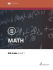### View a sample Math in Focus lesson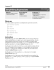### Dec-Jan - The Proper Group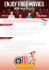### Someone to Help is Key Sinnissippi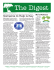### Expressions season brochure – July to December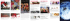### Roderick/Roland Tenorio Gems of Advice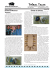### expressions live! - Expressions.org.nz